# Billard method

Jump to: navigation, search

for random covering

The Billard method was originally used to obtain a necessary condition for almost surely covering the circleby random intervals of given lengths(see Dvoretzky problem). Surprisingly, this necessary condition also turned out to be sufficient, not only in this case, but also in many extensions of the Dvoretzky problem. Unaware of this fact, P. Billard chose to give a weaker and more manageable condition, namely [a1]: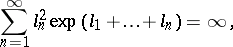(a1)

while the necessary and sufficient condition, stated by L. Shepp in 1972 is [a3]: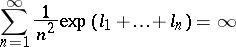(a2)

when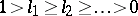(see Dvoretzky problem). Conditions (a1) and (a2) are quite close, but different; (a2) implies (a1), but (a1) does not imply (a2). Both are of interest when trying to cover the-dimensional torus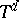almost surely by random translates of given convex sets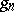with volumes(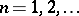). In that case, whatever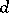may be, (a1) is necessary and (a2) is sufficient. The necessary and sufficient condition lies in between; it is (a2) whenand changes, tending to (a1), asincreases to infinity, at least if one restricts to homothetic simplices [a2].

The general setting for Billard's method is as follows:is a space, e.g.,,,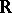, or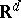;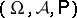is a probability space; the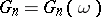(;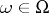) are random independent subsets of; andis a fixed subset of. One writesif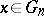and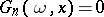otherwise. The problem of coveringalmost surely in such a way that each point belongs to infinitely many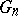reduces to verifying that the series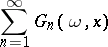diverges almost surely on. Billard's method is to consider the infinite product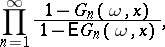where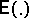denotes mathematical expectation. Ifcarries a probability measuresuch that the martingaleconverges in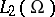, then the infinite product cannot vanish onalmost surely, and then finite covering cannot take place. This happens whenever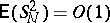, that is, when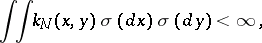where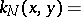Therefore,is not covered by infinitely manywhenevercarries a probability measure of bounded energy with respect to the kernels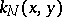.

In all cases of interest, this means thathas a strictly positive capacity with respect to a kernel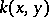(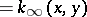). In the general setting, this is not a necessary and sufficient condition. However, it proves necessary and sufficient in the following cases:

1)and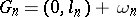, withindependent and Lebesgue-distributed on(the original Dvoretzky problem; here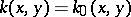and the condition reads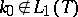);

2)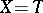,is a compact subset ofandas above;

3)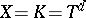and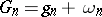, where theare homothetic simplices and the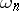are independent and Lebesgue-distributed on.

The Billard method gives a rough idea of the relation between random coverings and potential theory. To go further, more powerful methods are needed [a2] (see Fitzsimmons–Fristedt–Shepp theorem).

For additional references, see Dvoretzky problem.